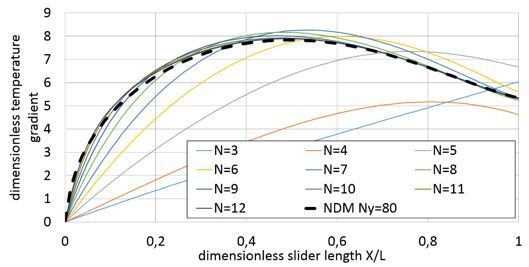Preprint Article Version 1 Preserved in Portico This version is not peer-reviewed

# About the Accuracy and the Grid Convergence of the Numerical Solution of the Energy Equation in Fluid Film Lubrication

Version 1 : Received: 13 September 2018 / Approved: 14 September 2018 / Online: 14 September 2018 (04:42:42 CEST)

A peer-reviewed article of this Preprint also exists.

Zhang, S.; Hassini, M.-A.; Arghir, M. Accuracy and Grid Convergence of the Numerical Solution of the Energy Equation in Fluid Film Lubrication: Application to the 1D Slider. Lubricants 2018, 6, 95. Zhang, S.; Hassini, M.-A.; Arghir, M. Accuracy and Grid Convergence of the Numerical Solution of the Energy Equation in Fluid Film Lubrication: Application to the 1D Slider. Lubricants 2018, 6, 95.

Journal reference: Lubricants 2018, 6, 95
DOI: 10.3390/lubricants6040095

## Abstract

The present work is focused on the numerical solution of the complete energy equation used in fluid film lubrication. The work was motivated by the fact the complete energy equation has no analytic solution that could be used for validations. Its accuracy and computation time are related to the employed numerical method and to the grid resolution. The natural discretization method (NDM) applied on different grids is systematically compared with the spectral method (the Lobatto Point Colocation Method or LPCM) with different polynomial degrees. A one dimensional inclined slider is used for the numerical tests and the energy equation is artificially decoupled from Reynolds. This approach enables to focus all the attention on the numerical solution of the energy equation. The results show that the LPCM is one or two orders of magnitudes more efficient than the NDM in terms of computation time. The energy equation is then coupled with Reynolds equation in a thermo-hydrodynamic analysis of the same 1D slider; the numerical results confirm again the efficiency of the LPCM. A thermo-hydrodynamic analysis of a two-lobe journal bearing is then presented as a practical application.## Subject Areas

energy equation; numerical solution; Lobatto point quadrature; Legendre polynomials

Views 0